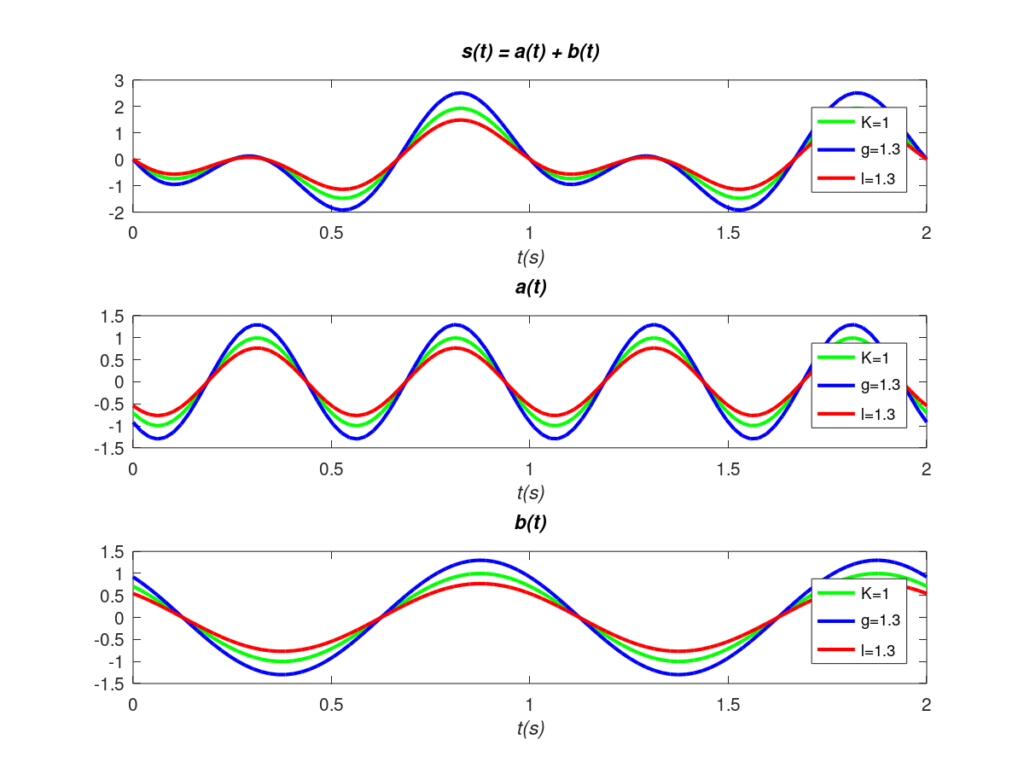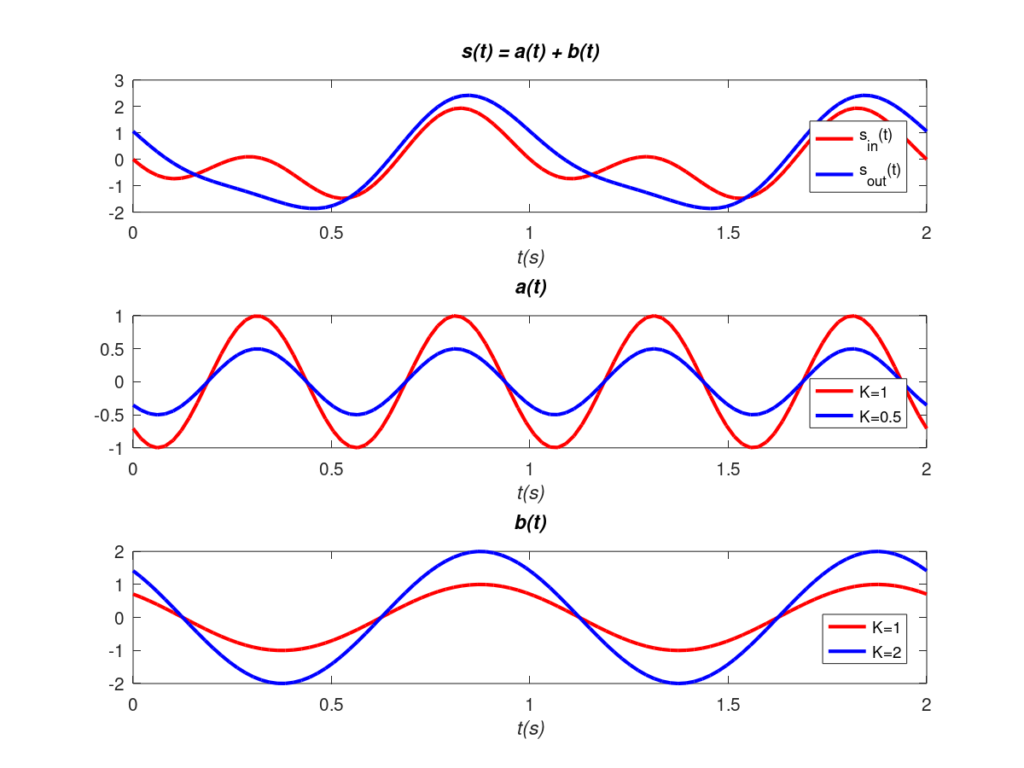# Amplitude Distortion: Explanation and Examples

Signals are composed of a sum of tones, each with its amplitude A, frequency f, and phase \footnotesize\varphi . Amplitude distortion refers to the change in appearance of the original signal due to disturbances produced directly on the amplitude of the original tones. This phenomenon is typically produced by transmission media or electronic components.

## Effects on Amplitude

The following mathematical model illustrates the direct effects of a transmission medium or component on the amplitude of the tones of a generic signal.

Let \footnotesize s_{in}(t) a signal composed of N tones, at the input of a transmission medium or component:

\begin{equation} s_{in}(t) = \sum_{i=1}^N{A_i}\cos(2{\pi}f_it\,+\,\varphi_i)\qquad i=1,2,3....,N \end{equation}

The direct effects of the medium or component on the amplitude of the original tones would result in the following output signal \footnotesize s_{out}(t):

\begin{equation} s_{out}(t) = \sum_{i=1}^N{K_i}{A_i}\cos(2{\pi}f_it\,+\,\varphi_i)\qquad i=1,2,3....,N \end{equation}

That is, the medium or component multiplies the amplitude of each frequency component \footnotesize A_i by a factor \footnotesize K_i.

## Constant Gain/Attenuation

When the parameter K is positive and constant for all frequencies, the resulting signal \footnotesize s_{out}(t) will have the same “aspect” as the original signal \footnotesize s_{in}(t). The only change is a proportionality factor, so that the information transmitted in the original signal is perfectly recoverable. In other words, no distortion occurs in this case. On the other hand, depending on the value of K, two different effects can occur.

Specifically, if all components are multiplied by a K value greater than one, \footnotesize s_{out}(t) will be an amplified replica of \footnotesize s_{in}(t). In this case g=K represents the component amplitude gain:

\begin{equation}\begin{split} s_{out}(t) &= \sum_{i=1}^N{g}{A_i}\cos(2{\pi}f_it\,+\,\varphi_i) \qquad i=1,2,3....,N \\ &= g\sum_{i=1}^N{A_i}\cos(2{\pi}f_it\,+\,\varphi_i) \\&=gs_{in}(t) \end{split}\end{equation}

Conversely, when K is less than one, the resulting signal will be an attenuated replica. In this case, the amplitude attenuation l is defined as l=1/K. Mathematically:

\begin{equation} s_{out}(t) =Ks_{in}(t)=\frac{1}{l}s_{in}(t) \end{equation}

An example illustrating these cases is shown below. The two frequency components, a(t) and b(t), of a generic signal, s(t), are multiplied or divided by the same factor. g for the amplitude gain case, and l for the amplitude attenuation case, respectively. Both the components and the resulting total signal are an equally amplified or attenuated replica of the originals. Therefore, no distortion is produced.Illustration of amplitude gain (blue) and amlitude attenuation (red) of a signal and its frequency components. No amplitude distortion is observed with respect to the original signal (green). (The original a(t) is defined by \footnotesize A_1=1, f_1=2, \varphi_1 = 3{\pi}/4 whereas the original b(t) is defined by \footnotesize A_2=1, f_2=1, \varphi_2 = {\pi}/4).

Note that if K is negative, in addition to the above, an inversion of the signal is also produced. In practice, an inversion does not produce distortion either, since the signal is still perfectly recoverable with another inversion.

## Amplitude Distortion

In contrast to the previous case, amplitude distortion occurs when the K factor is not uniform. In other words, \footnotesize K_i is different for at least two frequency components, and the resulting signal is not proportional to the input signal.

Mathematically, there is no constant value of K that proportionally relates input and output:

\begin{equation} s_{out}(t) = \sum_{i=1}^N{K_i}{A_i}\cos(2{\pi}f_it\,+\,\varphi_i)\; \cancel{=}\; Ks_{in}(t) \qquad i=1,2,3....,N \end{equation}

Amplitude distortion is illustrated with the following example. The starting point is the generic signal, \footnotesize s_{in}(t), composed of two frequency components, \footnotesize a(t) and \footnotesize b(t). On this occasion, different values of K are applied to both components (0.5 and 2 respectively). It can be seen that the resulting signal, \footnotesize s_{out}(t), no longer looks the same as the original because distortion, amplitude distortion, has occurred.Amplitude distortion due to non-uniform gains in the frequency components (original a(t) is defined by \footnotesize A_1=1, f_1=2, \varphi_1 = 3{\pi}/4 while original b(t) is defined by \footnotesize A_2=1, f_2=1, \varphi_2 = {\pi}/4. A K of 0.5 in a(t) and 2 in b(t) is applied).

(For a more formal analysis of the linear distortion, please refer to this link).

Bibliography
 Communication Systems, A. Bruce Carlson.

Subscription
If you liked this contribution, do not hesitate to subscribe to our newsletter: